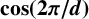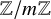### Real and complex fields (12Dxx)

• Article
• ##### FACTORING VARIANTS OF CHEBYSHEV POLYNOMIALS WITH MINIMAL POLYNOMIALS OF$\mathbf {cos}\boldsymbol {({2\pi }/{d})}$
• Bulletin of the Australian Mathematical Society, Volume 106, Issue 3

### General field theory (12Exx)

• Article
• ##### Decidability of the class of all the rings$\mathbb {Z}/m\mathbb {Z}$: A problem of Ax
• Forum of Mathematics, Sigma, Volume 11

• Article
• ##### FACTORING VARIANTS OF CHEBYSHEV POLYNOMIALS WITH MINIMAL POLYNOMIALS OF$\mathbf {cos}\boldsymbol {({2\pi }/{d})}$
• Bulletin of the Australian Mathematical Society, Volume 106, Issue 3

### Topological fields (12Jxx)

• Article
• ##### Decidability of the class of all the rings$\mathbb {Z}/m\mathbb {Z}$: A problem of Ax
• Forum of Mathematics, Sigma, Volume 11

### Connections with logic (12Lxx)

• Article
• ##### Decidability of the class of all the rings$\mathbb {Z}/m\mathbb {Z}$: A problem of Ax
• Forum of Mathematics, Sigma, Volume 11